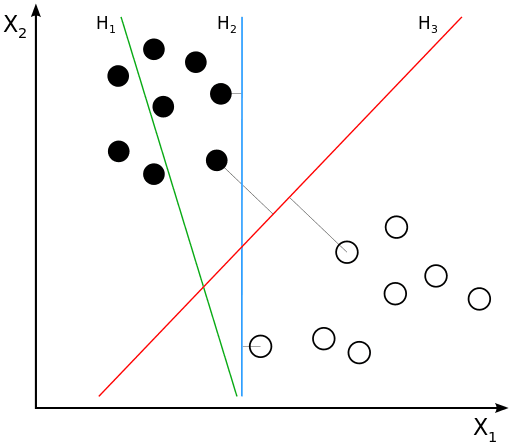# Support-vector machine from perspective of data scienceSrujan
Updated Jan 17, 2023
Support-vector machine from perspective of data science## Introduction

In machine learning, support-vector machines (SVMs, also support-vector networks) SVMs are one of the most robust prediction methods, being based on statistical learning frameworks or VC theory proposed by Vapnik (1982, 1995) and Chervonenkis (1974). Given a set of training examples, each marked as belonging to one of two categories, an SVM training algorithm builds a model that assigns new examples to one category or the other, making it a non-probabilistic binary linear classifier (although methods such as Platt scaling exist to use SVM in a probabilistic classification setting). SVM maps training examples to points in space so as to maximise the width of the gap between the two categories. New examples are then mapped into that same space and predicted to belong to a category based on which side of the gap they fall.

In addition to performing linear classification, SVMs can efficiently perform a non-linear classification using what is called the kernel trick, implicitly mapping their inputs into high-dimensional feature spaces.

When data are unlabelled, supervised learning is not possible, and an unsupervised learning approach is required, which attempts to find natural clustering of the data to groups, and then map new data to these formed groups. The support-vector clustering.

## Motivation

Classifying data is a common task in machine learning. Suppose some given data points each belong to one of two classes, and the goal is to decide which class a new data point will be in. In the case of support-vector machines, a data point is viewed as a p-dimensional vector (a list of p numbers), and we want to know whether we can separate such points with a (p-1)-dimensional hyperplane. This is called a linear classifier. There are many hyperplanes that might classify the data. One reasonable choice as the best hyperplane is the one that represents the largest separation, or margin, between the two classes. So we choose the hyperplane so that the distance from it to the nearest data point on each side is maximized. If such a hyperplane exists, it is known as the maximum-margin hyperplane and the linear classifier it defines is known as a maximum-margin classifier; or equivalently, the perceptron of optimal stability.## Applications

SVMs can be used to solve various real-world problems:

• SVMs are helpful in text and hypertext categorization, as their application can significantly reduce the need for labeled training instances in both the standard inductive and transductive settings. Some methods for shallow semantic parsing are based on support vector machines.
• Classification of images can also be performed using SVMs. Experimental results show that SVMs achieve significantly higher search accuracy than traditional query refinement schemes after just three to four rounds of relevance feedback. This is also true for image segmentation systems, including those using a modified version SVM that uses the privileged approach as suggested by Vapnik.
• Classification of satellite data like SAR data using supervised SVM.
• Hand-written characters can be recognized using SVM.
• The SVM algorithm has been widely applied in the biological and other sciences. They have been used to classify proteins with up to 90% of the compounds classified correctly. Permutation tests based on SVM weights have been suggested as a mechanism for interpretation of SVM models. Support-vector machine weights have also been used to interpret SVM models in the past. Posthoc interpretation of support-vector machine models in order to identify features used by the model to make predictions is a relatively new area of research with special significance in the biological sciences.